Working with BigInts

Operations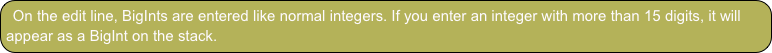Let’s enter a couple of BigInts and do operations on them.

Enter 12345678901234567

Enter 333444555666777888999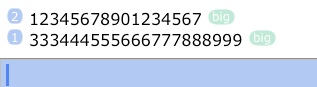Notice the “big” badge that BigInts carry.

Tap *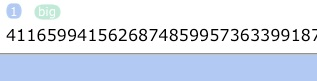The result value is a number so big that you have to side-scroll to see all the digits.

BigInts and “normal” numbers can be mixed.

Enter 3456

Tap /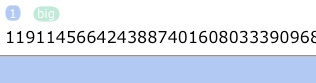(All functions work as expected, except division will always return a truncated integer result.)

Most of the functions in the Binary menu work with BigInts.

Go to the Binary menu. Tap hex (HEX)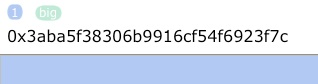The binary word size does not apply to BigInts and bit rotations (which rely on the word size) are not supported.

Tap → (NEXT). (Do this twice in Classic mode.)

Tap →right (SL)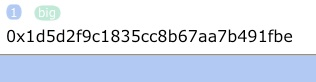Shifting in bit and byte units is supported.

As are the logic operators (and, or, xor, not).

Tap → (NEXT)

Enter 12345

Tap and (AND)Although this is usually done automatically done for you, there’s two functions that allow you to explicitly convert to (and from) BigInts: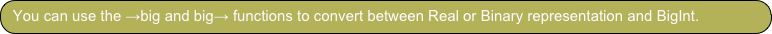Tap big→Notice that the “big” badge disappeared.

Converting from BigInt makes the number is useful to make the number usable in for all functions/operators that expect real or binary values.

Tap →bigThe “big” badge reappeared and the number is a BigInt again.

In addition to typing in a big integer, or tapping →big, the third way to obtain a BigInt is as the result of certain function calls. A few functions that normally return real numbers will return BigInts for large arguments, for example.

Type “100!” (w/o quotes)The “!” operator invokes the factorial function and returns a BigInt result for arguments greater than 100.

Enter.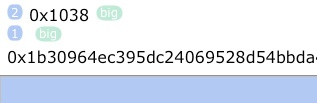(The result is once again a very large number. See Visualizing 987! for a fun tutorial to make a long number “visible”.)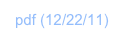OverviewMost basic operators (“+”, “-”, “*”, “/”, “^”), most functions in the Binary menu, along with some select functions from the Real menu, work with big integers. (For details, see the Functions list.)

You can use big integers in RPL programs and expressions, either in literal form (i.e., as something like “934629387468264782368234234”) or in variables.

This tutorial shows you how to enter and do some basic operations using BigInts. A comprehensive example of the use of BigInts in given in “The Fib Triangle”.

While BigInts only hold integer values, they can be used to emulate or compute “big float” numbers. An example of this is given at the end of this tutorial. ND1 also supports BigFloats, for arbitrary-precision real numbers.

Computing with BigInts

You can use BigInts in your RPL and JavaScript programs.

In RPL, you can use BigInts just like normal numbers (while keeping in mind the limits on its use; you cannot use them with most real-valued functions, such as sin (SIN), for example). Use →big (or the equivalent, but easier to type, function name “toBig”) to convert a loop counter to a BigInt, if necessary.

Examples for use of BigInts in RPL can be found in the BigInt, Project Euler, and Examples shared folders. (See, for example ”The Fib Triangle”.)

In JavaScript, you cannot use BigInts like a normal number.

Instead you need to write code looking like this:

function(nDigits) {

var numerator = BigNum["pow"](BigNum.fromNumber(10), BigNum.fromNumber(nDigits));

var bigE = BigNum["+"](numerator, numerator); // first two terms

var factorial = BigInteger.ONE;

var counter = BigInteger.ONE;

var seriesVal;

do {

factorial = BigNum["*"](factorial, counter = BigNum.incr(counter));

seriesVal = BigNum["/"](numerator, factorial);

bigE = BigNum["+"](bigE, seriesVal);

}

while (BigNum[">"](seriesVal, BigInteger.ZERO));

return new BigNumber("2." + BigNum.toString(bigE).slice(1,-2), nDigits);

}

The code shown is taken from the AP Constants public folder and computes a BigFloat for the number “e” for a desired number of digits.

See Custom Data Types for a discussion of the BigInt data type.